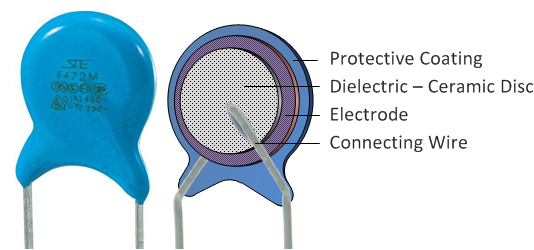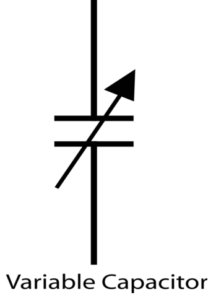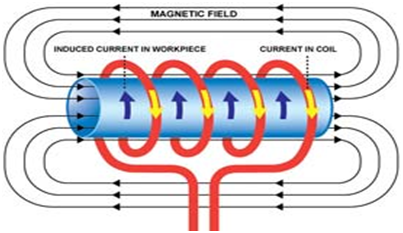# Passive Components – Capacitors, Inductors### Capacitors:

The capacitor is the linear passive component which is used to store the electric charge. It will have the pair of electrodes between which have the insulating dielectric material.

Stored charge Q= CV (C= capacitive reactance, V= applied voltage)

Current through capacitor I= C dv/dt

### Types

Fixed capacitors(these offers the fixed reactance to flow the current)

• Ceramic Capacitor(consists of the ceramic plates made by silver)• Electrolyte Capacitor• (These are polarized and used for the high value of capacitance)Variable Capacitors(They offer capacitance which can be varied by varying the distance between the plates. They can be air gap capacitors or vacuum capacitors.)

### Inductors:An inductor stores energy in form of a magnetic field. It generally consists of a conductor coil, which offers a resistance to the applied voltage. It works on the basic principle of Faraday’s law of inductance, according to which a magnetic field is created when current flows through the wire and the electromotive force developed opposes the applied voltage.stored energy E = LI^2. ( L is the inductance measured in Henries, I is the current flowing through it.)Likes:
0 0
Views:
51
Article Tags:
Article Categories: